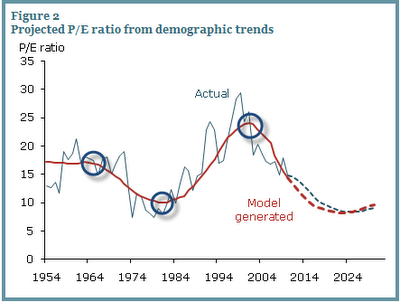Select Page

“The barometric needle has switched over to the bear side”

Posted by Mike Shedlock - Richard Russell CommentDemographic Headwinds for US Stock Prices This evidence suggests that U.S. equity values are closely related to the age distribution of the population. Since demographic trends are largely predictable, we can forecast the path that the P/E ratio is likely to follow in the next few decades based on the predicted M/O ratio. Figure 2 compares the actual and model-implied P/E ratios for the sample period ending in 2010. We calculate the path for the model-implied P/E during the sample period by feeding in actual M/O ratios. We call the long-run path of the P/E ratio predicted by the model the “potential P/E ratio” and designate it P/E*. Figure 2 shows that the P/E* (red dashed line) is highly correlated with actual P/E during the sample period.….read more and view Charts HERE